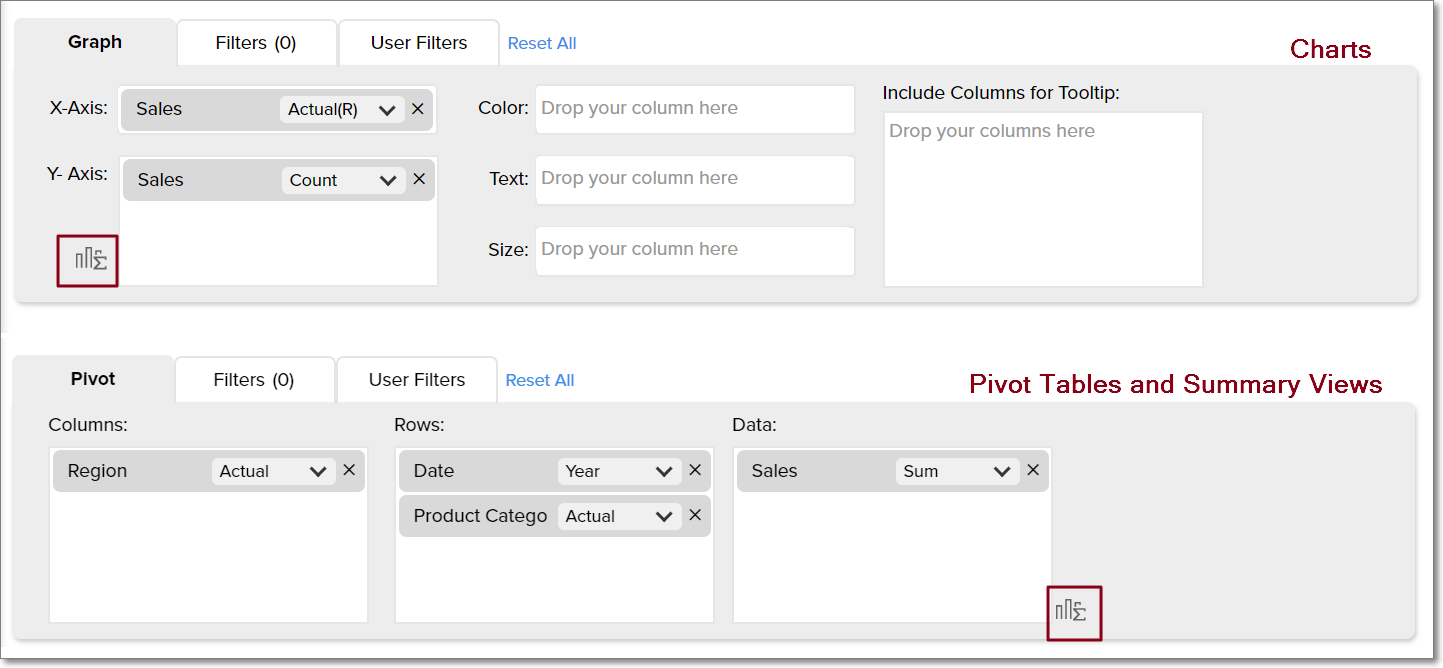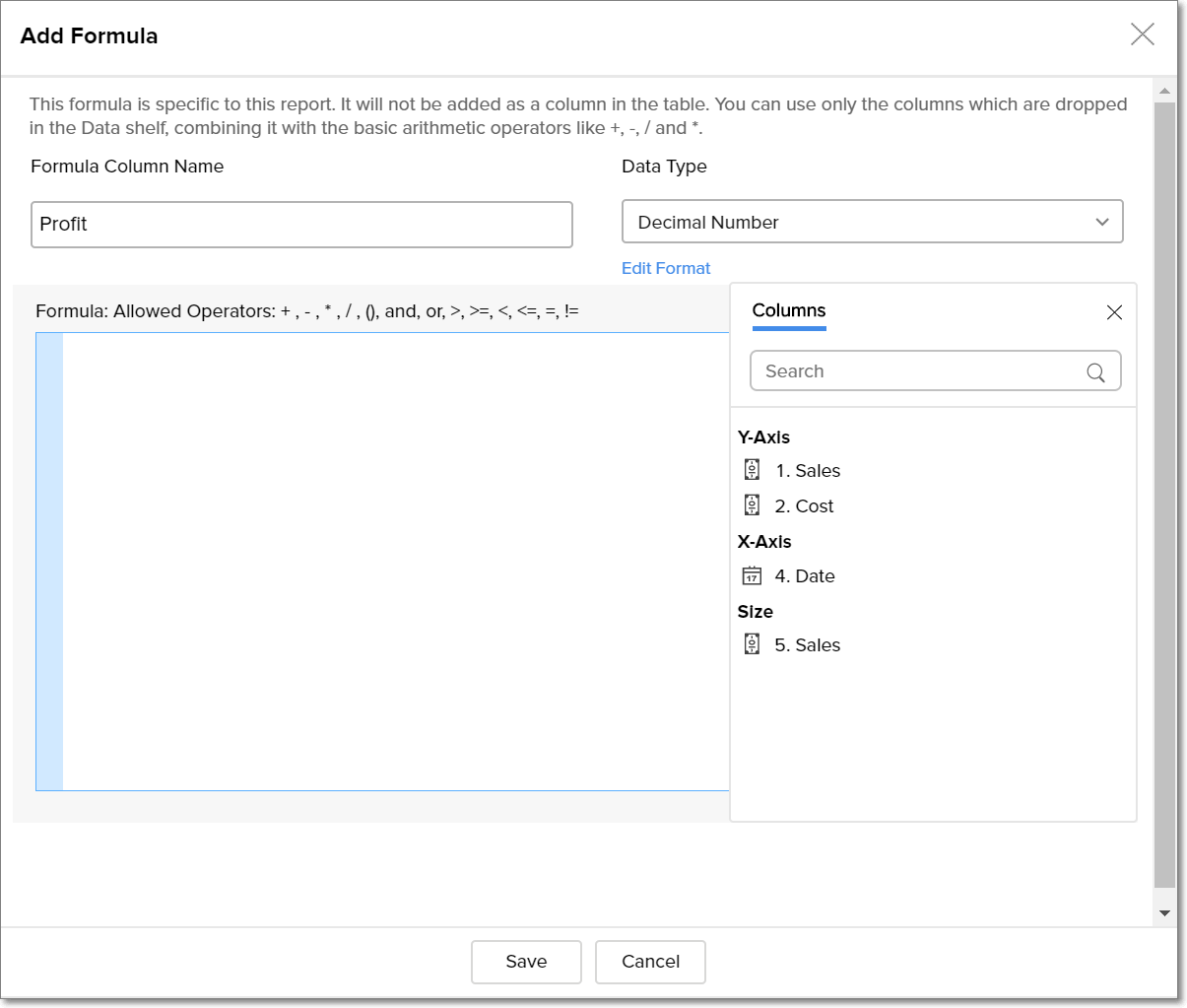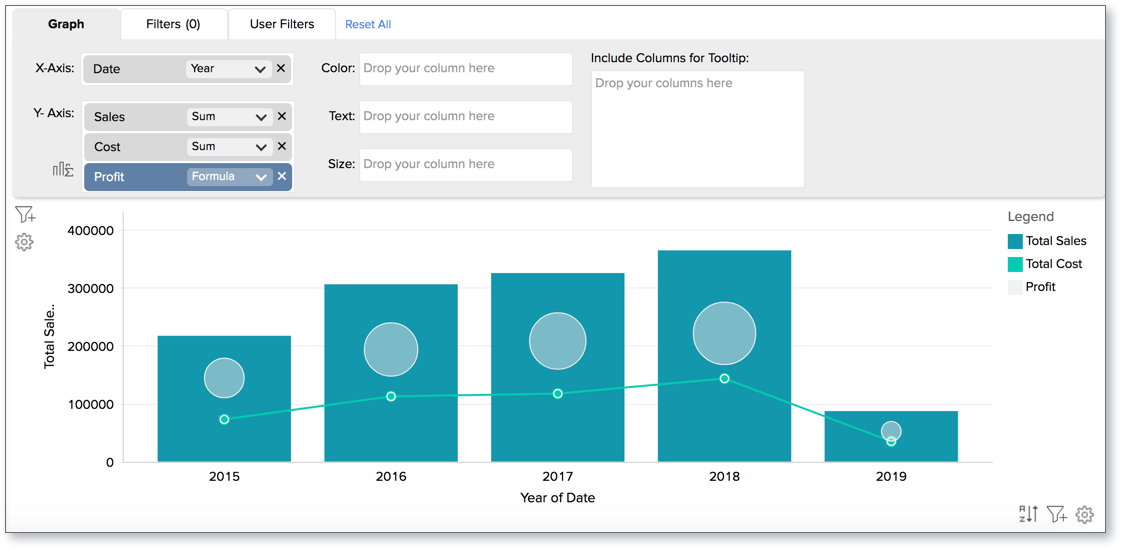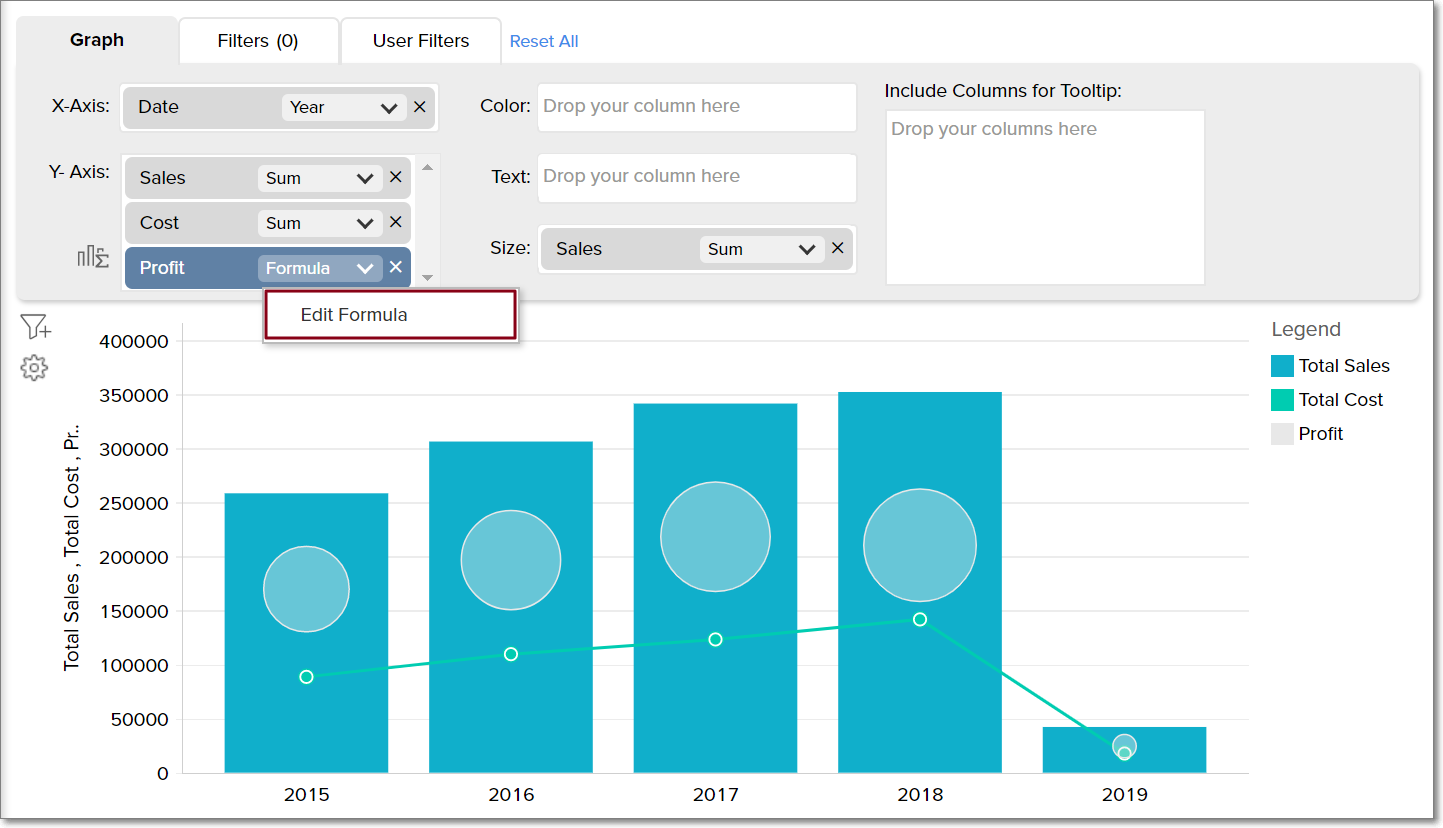# Report Formula

Analytics Plus provides an option called Report Formula, which enables you to perform arithmetic calculations over the metrics used in a particular report.

### 1. What is a report formula?

Report formula is a form of calculated metric that is specific to a particular report. While Formula columns and Aggregate Formulas are applied and associated to a data table, report formulas are applicable to only the report they are created over. Using this option, you can perform basic operations on the columns dropped in the Y-axis shelf for chart views, and in the Data shelf for pivot and summary views.

### 2. Are report formulas supported in all report types?

Report formulas are only supported for the following report types:

• Chart
• Pivot Table
• Summary View

### 3. How do I create a report formula?

Follow the below steps to create a report formula.

1. Open the report in Edit mode and click the formula icon.2. In the Add Formula page that appears, provide the formula column name and select the data type.3. Specify the required formula in the editor. You can perform basic arithmetic, logical and comparison operations using the metrics in the report. The allowed operators are: + , - , * , / , (), >, <, >=, <=, =, !=, AND, OR and NULL.

4. Click Save. The created Report Formula will be added as a metric in the report.Note: Report formulas will not be added as columns to the base table.

### 4. How do I edit report formulas?

Follow the steps below to edit a report formula:

1. Open the report in Edit mode.

2. Navigate to the report formula metric and click Edit Formula.3. The formula dialog will open. Edit the formula as required.

### 5. Can I use the report formulas of one report in another?

No. Formulas created over a report are specific to that report alone, and cannot be used in other reports. To create formulas that can be reused in several reports, create the formula in the base table. Click here for more information.